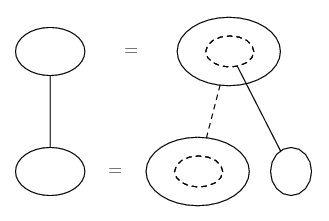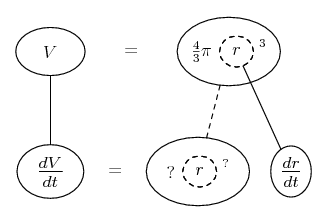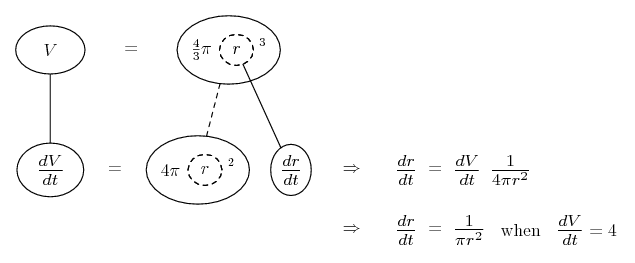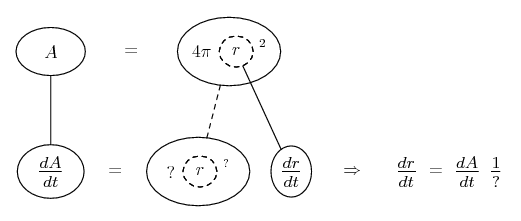# Thread: rate of change problem

1. ## rate of change problem

The volume of a spherical balloon is increasing at a rate of 4 cubic meters per minute. How fast is the surface area increasing when the radius is 3 meters?

2. Just in case a picture helps...

Related rates nearly always depend on the chain rule, so you might want to try filling up this pattern...... where straight continuous lines differentiate downwards (integrate up) with respect to the main variable (in this case time), and the straight dashed line similarly but with respect to the dashed balloon expression (the inner function of the composite which is subject to the chain rule).

So what have we got here? V, dV/dt, r, and the formula for V in terms of r...So differentiate with respect to r...OK, so what about surface area? Well, we can do the same for that...Now you've got two expressions for dr/dt, so equate them and solve for dA/dt.

__________________________________________

Don't integrate - balloontegrate!

Balloon Calculus: Gallery

Balloon Calculus Drawing with LaTeX and Asymptote!

#### Search Tags

change, problem, rate